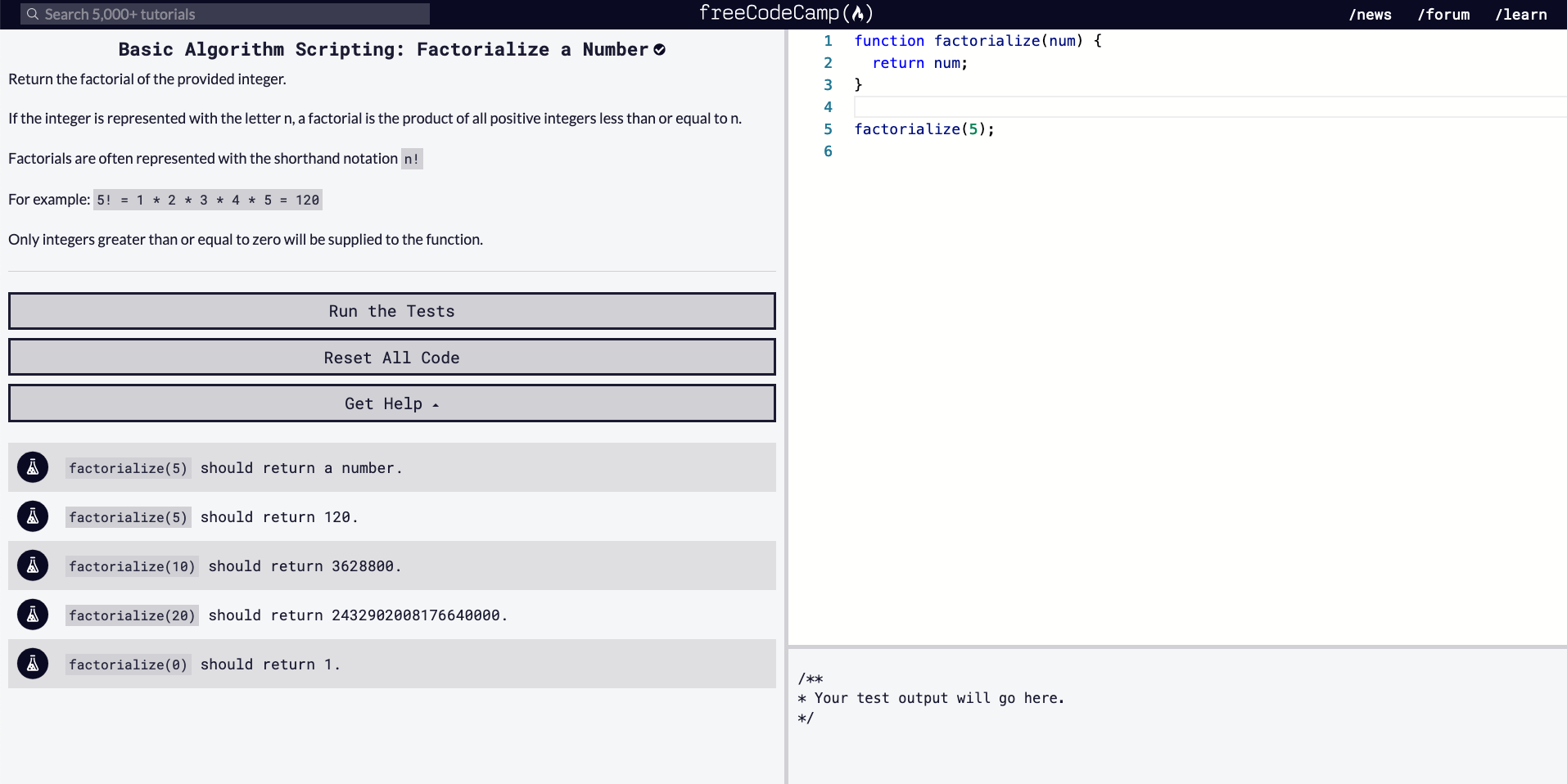# FCC Algorithm Challenges / Factorialize a Number

### 4/27/2020``````function factorialize(num) {
return num;
}

factorialize(5);

/// TESTS
factorialize(5) should return a number.
factorialize(5) should return 120.
factorialize(10) should return 3628800.
factorialize(20) should return 2432902008176640000.
factorialize(0) should return 1.
``````

Above is the starter code provided for the challenge, "Factorialize a Number".

Our goal is to write a function that to take the input of a number and return its factorial (a product). Let's think this through. Here is how I would aim to solve this problem.

## Method

• Read the instructions first. Make sure you understand what it being asked of you.
• Read the starter code. Go line by line, just making sure you know what is going on initially.
• Have a look at the tests. If the problem isn't clear to you, looking at the tests might give you an inclination of what kind of output you should aim for (i.e. instead of returning an array, maybe the problem is only asking for an index within the array).
2. Think & Write

Now that you've read through the instructions, starter code, and tests, it's time to analyze what to do and in what order. It may be handy to write out pseudocode.

3. Code

Once you've thought about what you'd like to do, and in what order, start to convert your pseudocode into JavaScript code.

There's been too many times where I've tried to jump write into writing the code without thinking it through (in projects and coding challenges). That will leave you testing it way too many times, creating unnecessary variables, and running into more problems then you need to handle. If I try to follow the above method, it leaves me with a more clear mind of what I'm doing and hopefully writing some DRY code. Let's try to solve this problem now.

## Thoughts

• Factorials can be a complicated idea
• It seems we have to return the product of the input number, `num` which multiples itself by all other positive smaller integers
• Meaning `num = 5`, so 5 * 4 * 3 * 2 * 1
• There is a statement in the problem, mentioning, only integers greater than or equal to zero will be supplied to the function
• We'll have to check if num is bigger than 0, if it is 0 or less, we we will check with an if statement and return 1;
• We can use a while loop instead of a for loop for this problem and reduce `num - 1` after each loop of multiplication
• Make sure to return the `total` (the product variable)

** Afterthought, whoops I could have used and practiced using a recursive function to solve.

## Solution

Some Pseudocode

``````function factorialize(num) {
set up total (we will use this store our product)

check if num is bigger than 0
if not bigger, return the 1

while num is greater than 0
loop through num times
total = total * num
num - 1

return the product (total)
}
``````
**[SPOILER: SOLUTION TO CODE BELOW]** ```javascript function factorialize(num) { let total = 1;

while (num > 0) { total = total * num; num--; }

``````This problem can also be solved by using a recursive function.

</details>